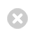Close

MPHY0021: Research Software Engineering With Python

Home## Recap: Understanding the "Greengraph" Example¶

We now know enough to understand everything we did in the initial example chapter on the "Greengraph" (notebook). Go back to that part of the notes, and re-read the code.

Now, we can even write it up into a class, and save it as a module. Remember that it is generally a better idea to create files in an editor or integrated development environment (IDE) rather than through the notebook!

### Classes for Greengraph¶

The original example was written as a collection of functions. Alternatively, we can rewrite it in an object-oriented style, using classes to group related functionality.

In :
%%bash
mkdir -p greengraph  # Create the folder for the module (on mac or linux)

In :
%%writefile greengraph/graph.py
import numpy as np
import geopy
from .map import Map

class Greengraph(object):
def __init__(self, start, end):
self.start = start
self.end = end
self.geocoder = geopy.geocoders.Yandex(lang="en_US")

def geolocate(self, place):
return self.geocoder.geocode(place, exactly_one=False)

def location_sequence(self, start, end, steps):
lats = np.linspace(start, end, steps)
longs = np.linspace(start, end, steps)
return np.vstack([lats, longs]).transpose()

def green_between(self, steps):
return [Map(*location).count_green()
for location in self.location_sequence(
self.geolocate(self.start),
self.geolocate(self.end),
steps)]

Writing greengraph/graph.py

In :
%%writefile greengraph/map.py

import numpy as np
from io import BytesIO
import imageio as img
import requests

class Map(object):
def __init__(self, lat, long, satellite=True, zoom=10,
size=(400, 400), sensor=False):
base = "https://static-maps.yandex.ru/1.x/?"

params = dict(
z=zoom,
size=str(size) + "," + str(size),
ll=str(long) + "," + str(lat),
l="sat" if satellite else "map",
lang="en_US"
)

self.image = requests.get(
base, params=params).content  # Fetch our PNG image data
content = BytesIO(self.image)
self.pixels = img.imread(content) # Parse our PNG image as a numpy array

def green(self, threshold):
# Use NumPy to build an element-by-element logical array
greener_than_red = self.pixels[:, :, 1] > threshold * self.pixels[:, :, 0]
greener_than_blue = self.pixels[:, :, 1] > threshold * self.pixels[:, :, 2]
green = np.logical_and(greener_than_red, greener_than_blue)
return green

def count_green(self, threshold=1.1):
return np.sum(self.green(threshold))

def show_green(data, threshold=1.1):
green = self.green(threshold)
out = green[:, :, np.newaxis] * array([0, 1, 0])[np.newaxis, np.newaxis, :]
buffer = BytesIO()
result = img.imwrite(buffer, out, format='png')
return buffer.getvalue()

Writing greengraph/map.py

In :
%%writefile greengraph/__init__.py
from .graph import Greengraph

Writing greengraph/__init__.py


### Invoking our code and making a plot¶

In :
from matplotlib import pyplot as plt
from greengraph import Greengraph
%matplotlib inline

mygraph = Greengraph('New York', 'Chicago')
data = mygraph.green_between(20)

---------------------------------------------------------------------------
TypeError                                 Traceback (most recent call last)
/tmp/ipykernel_9673/2179771185.py in <module>
3 get_ipython().run_line_magic('matplotlib', 'inline')
4
----> 5 mygraph = Greengraph('New York', 'Chicago')
6 data = mygraph.green_between(20)

~/work/rsd-engineeringcourse/rsd-engineeringcourse/ch01data/greengraph/graph.py in __init__(self, start, end)
8         self.start = start
9         self.end = end
---> 10         self.geocoder = geopy.geocoders.Yandex(lang="en_US")
11
12     def geolocate(self, place):

TypeError: __init__() got an unexpected keyword argument 'lang'
In :
plt.plot(data)

---------------------------------------------------------------------------
NameError                                 Traceback (most recent call last)
/tmp/ipykernel_9673/4078587883.py in <module>
----> 1 plt.plot(data)

NameError: name 'data' is not defined